International Journal of Energy and Power Engineering
Volume 4, Issue 4, August 2015, Pages: 216-226

Optimum Design of Penstock for Hydro Projects

Singhal M. K., Arun Kumar

AHEC, Indian Institute of Technology, Roorkee, India(Singhal M. K.)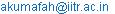(Arun K.)

Singhal M. K., Arun Kumar. Optimum Design of Penstock for Hydro Projects. International Journal of Energy and Power Engineering. Vol. 4, No. 4, 2015, pp. 216-226. doi: 10.11648/j.ijepe.20150404.14

Abstract: Penstock, a closed conduit, is an important component of hydropower projects. Various methods are available for optimum design of penstock. These methods are either based on empirical relations or derived analytically by optimizing the friction loss in the penstock. These formulae produce different values of penstock diameter for same site. In this study, formulae available for penstock design have been compared to review their suitability. A new method has been developed for the optimum design of penstock based on minimizing the total head loss comprising of friction and other losses. By using new developed method, diameter and annual cost of penstocks for few Hydro Electric plants of varying capacity have been worked out and reduction in annual cost of penstocks have been found in comparison to penstock cost for these projects.

Keywords: Friction Losses, Total Head Losses, Annualized Penstock Cost, Optimum Diameter of Penstock

1. Introduction

Hydropower, a renewable and mature energy source utilizes water from higher to lower altitude to generate power. Hydro Power is one of the proven, predictable and cost effective sources of renewable energy. Hydropower system (Fig 1) comprises of hydro source, diversion/storage system, water conductor system (channel/tunnel/penstock), power house building, generating and control equipment. Penstock is a conduit or tunnel connecting a reservoir/forebay to hydro turbine housed in powerhouse building for power generation. It withstands the hydraulic pressure of water under static as well as dynamic condition. It contains the closing devices (gates /valves) at the starting (just after reservoir/forebay) and at the tail end just before turbine to control the flow in the penstock. The penstock material may be mild steel, glass reinforced plastic (GRP), reinforced cement concrete (RCC), wood stave, cast iron and high density polyethylene (HDPE) etc. However, in the most of the cases, mild steel has been used for penstock since long due to wider applicability and availability. The penstock cost contributes an appreciable percentage towards the total civil works cost of the hydroelectric project. By optimizing the penstock diameter, maximum energy generation can be obtained at minimum cost.

The aim of present study is to develop the new relation for the optimum design of penstock by considering all types of losses in the penstock.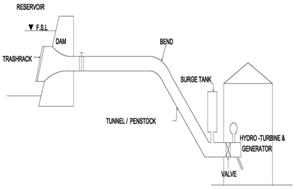Fig. 1. Penstock and Hydropower system schematic layout.

2. Relations Available for Optimized Design of Penstock

Various researchers have proposed the methodology and relations for the optimum design of penstock. These relations are either empirical relations developed by analyzing and correlating statistical data of existing/installed projects designed as per past practice or derived analytically by minimizing annual penstock cost considering friction loss. The available analytical relations considered only friction loss whereas in addition to friction loss, there are other losses such as losses at specials (bends, transition piece, tri/bifurcation etc.), losses at valves and inlet which are not considered while optimizing the penstock design.

Annual penstock cost comprises of investment cost on penstock material, excavation for penstock, concreting, installation, operation, maintenance. depreciation and cost of energy loss caused by head loss.

The available empirical relations can be grouped in different categories based on parameters used to determine the optimum penstock diameter.

2.1. Empirical Relations Based on Penstock Discharge (Q)

Warnick et al. (1984) developed formula for optimum diameter of penstock pipe (De) for small hydro projects in terms of rated discharge (Q) as shown in eqn. (1)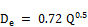(1)

2.2. Empirical Relations Based on Project Installed Capacity (P) and Rated Head (Hr)

Bier (1945), Sarkaria (1979) and Moffat et al. (1990) have developed the formulae for estimation of economical diameter of penstock in terms of installed capacity (P) and rated head (Hr). Warnick et al. (1984) developed formula for optimum diameter of penstock pipe for large hydroelectric projects having rated head between 60 m to 315 m and power capacities ranging from 154 MW to 730 MW. These relations are shown in eqn. (2) to eqn. (5)

Bier (1945) relation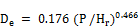(2)

Sarkaria (1979) relation(3)

Warnick et al. (1984) relation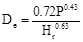(4)

Moffat et al. (1990) relation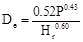(5)

2.3. Empirical Relations Based on Penstock Discharge (Q) and Rated Head (Hr)

Voetsch and Fresen (1938), USBR (1986), ASCE (1993) and Fahlbusch (1987) have developed the relations for economic penstock diameter in terms of rated discharge and rated head. These relations are shown as eqn. (6) to eqn. (9)

Voetsch and Fresen (1938) relation

This relation can be used for penstock having discharge more than 0.56 m3/s . As per this method, coefficient (K) is computed by using eqn.(6)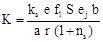(6)

The loss factor () is obtained on the basis of plant load factor (pf) from Graph A (16). K is used to select value of coefficient B and coefficient D’ corresponding to penstock discharge and gross head from graph B and graph C respectively. Optimize diameter for penstock is calculated as De = B x D’

USBR (1986) relation(7)

After simplification, eqn. (7) may be written as shown in eqn. (8)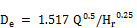(8)

Fahlbusch (1987) relation(9)

ASCE (1993) relation

The relation given by ASCE is shown in eqn. (10).This relation is in FPS system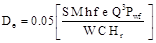(10)

Present worth factor (Pwf ) is computed from eqn. (11 )(11)

2.4. Analytical Relation for Optimize Design of Penstock

Analytical relation available for optimum diameter of penstock in Indian Standard (IS) 11625, is based on minimizing the total annual expenditure on penstock considering only friction loss. The relation is shown as eqn. (12).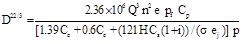(12)

3. Comparative Study of Various Relations Available for Optimum Diameter of Penstock

In this study, the comparative study of relations available for optimum diameter of penstock has been done by calculating penstock diameter for few Hydro Electric (HE) projects in India by these methods. The features of HE projects analyzed are shown in Table 1. For projects with higher gross head, the effect of head on computing penstock diameter becomes significant. Therefore, to compare the results of these relations, the projects are grouped in three categories based on gross head. The projects having gross head up to 100 m are grouped in Category A, 100 m to 200 m in category B and above 100 m in category C. The penstock diameter computed for these projects have been shown in Table 2. From the analysis of results shown in this table, it may be seen that the values of diameters obtained from these relations are different. The results have been compared in Fig. 2, Fig. 3 and Fig. 4 for projects in category A, category B and category C respectively. From these figures it may be seen that ASCE and Warnick relations generally provide the penstock diameters on higher side while Bier and USBR relations provide the penstock diameter on lower side.

Table 1. Features of HE projects Analyzed in the Study.

 Sl. No. Project Capacity (kW) Discharge (m3/s) Penstock Length (m) Gross Head (m) Category A Projects 1 Dugtu 25 0.17 360 31.25 2 Gaundar 100 0.38 105 49.16 3 Kuti 50 0.38 200 53.45 4 Kotijhala 200 0.48 120 80.60 5 Wachham 500 1.38 120 48.94 6 Debra 1500 1.84 138 95.35 7 Dhera 1500 2.12 170 85.20 8 Gaj 1500 4.88 121 38.44 9 Nyikgong 13000 23.75 156 78.00 10 Kamlang 24900 68.02 2260 44.92 Category B Projects 11 Baram 1000 1.90 700 127.50 13 Divri 3200 3.06 191 118.42 14 Sarbari-ii 5400 3.65 365 191.37 12 Keyi 23000 20.50 1653 127.54 15 Thru 60000 37.18 8190 191.57 16 Phunchung 45000 37.46 4184 149.00 Category C Projects 17 Jirah 4000 1.34 870 380.00 18 Ditchi 2500 1.45 453 204.35 19 Luni-II 5000 1.66 960 358.40 20 Luni-III 5000 1.70 1250 363.18 21 Pemashelpu 81000 34.07 350 289.00

Table 2. Penstock Diameter obtained using Various available Methods.

 Sl. No. Project Penstock dia based on analytical method (IS) (m) Penstock Diameter (m) based on empirical Methods Moffat Warnick Sarkaria Fahlbusch USBR Bier ASCE Voetsch & Fresen Category A Projects 1 Dugtu 0.38 0.27 0.29 0.31 0.33 0.27 0.16 0.53 - 2 Gaundar 0.53 0.37 0.44 0.41 0.45 0.35 0.25 0.70 - 3 Kuti 0.53 0.26 0.44 0.29 0.45 0.35 0.17 0.69 - 4 Kotijhala 0.58 0.37 0.50 0.40 0.48 0.35 0.27 0.73 - 5 Wachham 0.91 0.73 0.85 0.82 0.81 0.68 0.52 1.23 0.91 6 Debra 1.01 0.79 0.98 0.86 0.85 0.66 0.64 1.26 0.95 7 Dhera 1.07 0.84 1.05 0.92 0.92 0.73 0.67 1.37 1.03 8 Gaj 1.55 1.36 1.59 1.55 1.48 1.35 0.97 2.19 1.64 9 Nyikgong 2.82 2.24 3.51 2.47 2.76 2.49 1.91 3.90 2.86 10 Kamlang 4.64 4.34 5.94 4.92 4.79 4.94 3.48 6.62 4.92 Category B Projects 11 Baram 1.00 0.56 0.99 0.60 0.84 0.63 0.46 1.23 0.88 13 Divri 1.22 0.96 1.26 1.03 1.05 0.81 0.82 1.52 1.14 14 Sarbari-ii 1.27 0.90 1.38 0.95 1.07 0.78 0.84 1.54 1.07 12 Keyi 2.68 2.18 3.26 2.34 2.45 2.06 2.01 3.41 2.50 15 Thru 3.34 2.64 4.39 2.79 3.06 2.54 2.66 4.15 2.90 16 Phunchung 3.41 2.67 4.41 2.84 3.16 2.69 2.58 4.32 3.05 Category C Projects 17 Jirah 0.79 0.53 0.83 0.53 0.63 0.40 0.53 0.91 0.67 18 Ditchi 0.86 0.62 0.87 0.65 0.70 0.48 0.57 1.02 0.80 19 Luni-II 0.87 0.60 0.93 0.61 0.70 0.45 0.61 1.00 0.68 20 Luni-III 0.87 0.60 0.94 0.61 0.70 0.46 0.60 1.01 0.76 21 Pemashelpu 3.11 2.25 4.20 2.31 2.78 2.15 2.44 3.77 2.74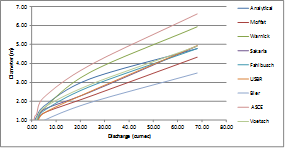Fig. 2. Relation between Penstock diameter and discharge for category A projects.Fig. 3. Relation between Penstock diameter and discharge for category B projects.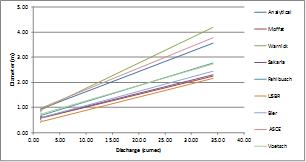Fig. 4. Relation between Penstock diameter and discharge for category C projects.

4. Effect of Head Loss on the Computation of Optimum Diameter of Penstock

The optimum diameter of penstock is computed based on minimizing the total annualized cost of the penstock comprising of annualized expenditure on penstock and annual loss of revenue for energy loss caused by head losses in the pipe. For smaller diameter penstock, the expenditure on penstock will be less but, this will have higher head loss leading to higher energy and revenue loss. Similarly, the larger diameter penstock will have less head loss resulting in more energy generation and higher revenue but, will have more installation cost leading to higher annual cost of penstock. Therefore, head loss in penstock effects the determination of optimum diameter of penstock.

The head loss in penstock comprises of friction loss (hf) due to resistance offered by penstock inner surface and other losses. The sources of other head losses (h0) are specials comprising of intake provided at the entry of penstock with reservoir to allow smooth entry of discharge into penstock, control equipment (gate or valve) provided with penstock outside the reservoir/forebay to control the penstock discharge, bends provided at each location of change in penstock alignment, bifurcation (wye) piece provided at the end of penstock to connect it with its branches (one each for each unit), inlet valve  provided at the end of each penstock branch to control the flow entering the turbine and transition piece (expansion or reducer) provided before or after inlet valve to connect it with penstock branch or with turbine.

The head loss also occurs at trash rack provided in front of penstock intake which checks the entry of floating and other material into the penstock. As the head loss at trash rack is not dependent on the flow velocity in the penstock and is not considered for the optimum design of penstock.

4.1. Determination of Friction Loss (hf) in Penstock

The relation for determination of friction loss in penstock was first given by Chezy (1775). Subsequently, improved relations were given by various researchers. Some of these relations are for laminar flow having Reynold number (Re) less than 2000, some are for transition flow having Re in between 2000 and 4000 and some are for turbulent flow having Re more than 4000.

Poiseuille (1841) developed the relation for laminar flow. Hazen Williams (1902) and Blasius (1913) developed the relations for turbulent flow. The flow in penstock shall be turbulent flow as penstock with very small diameter like 0.1 m and flow velocity as 2.0 m/sec shall have Re as 2 x 105 which is in the range of turbulent flow. The relation developed by Hazen Williams is not much used for penstock as this relation can be effectively used for the flow velocity up to 1.0 m/sec which is much less than the flow velocity in penstock. The relation developed by Blasius is also not applicable for penstock flow as relation given by him is applicable for flow having Re up to 1´105 which is less than the Re of flow in penstock. The relations developed by Darcy-Weisbach (1850), Manning (1891) and Scobey (1930) are the most commonly used relations to determine the friction loss in penstock.

4.1.1. Friction Loss as Per Manning Relation

Manning relation computes the friction loss in pipe flow in terms of roughness coefficient, flow velocity, penstock length and hydraulic radius as shown in equation (13).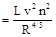(13)

In Manning equation, roughness coefficient is not dimensionless which lead to variation in Manning roughness coefficient for different penstock diameters under same flow conditions. Further, in Manning relation, flow characteristics are not accounted. The Manning roughness coefficient for steel pipe is taken between 0.008 to 0.012. By taking v = 4 Q/(pD2) and R = D/4, eqn. (13) may be simplified as shown in eqn. (14)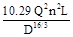(14)

4.1.2. Friction Loss as Per Darcy Weisbach Relation

Darcy – Weisbach relation computes the friction loss in pipe flow in terms of friction coefficient and other parameters as shown in eqn. (15)(15)

By taking v = 4 Q/(pD2) and g = 9.81, eqn. (15) may be simplified as shown in eqn. (16)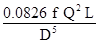(16)

To determine friction coefficient in Darcy Weisbach equation, Nikuradse (1933) carried out experiments on uniformly roughened pipes and developed curves between friction coefficient and Re. Karman Prandtl (1932) developed the empirical relation for these curves. These curves and relation are having limited application as in practice, the roughness in pipes is not uniform but irregular and wavy.

Colebrook and White (1937) developed the relation between Re and f by carrying out experiments on commercially available pipes considering the effect of height of roughness in pipes. The equation given by them required various trials for solution as their equation was implicit. Moody’s (1944) developed the curves corresponding to different values of relative roughness (k/D) for giving direct values of friction coefficient based on Colebrook White relation. Swami and Jain (1976) developed the explicit relation to determine the friction coefficient in terms of Re and relative roughness height (k/D) based on Colebrook and White relation as per eqn. 17.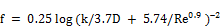(17)

4.1.3. Friction loss as per Scobey Relation

Scobey relation computes the friction loss in terms of friction coefficient and other parameters as shown in eqn. (18). The value of this coefficient is taken in between 0.32-0.36 with average value as 0.34.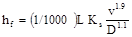(18)

The relation shown in eqn. (18) is in FPS system. This relation has been modified in MKS system and shown as eqn. (19)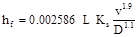(19)

4.2. Development of Relations Between Various Friction Coefficients

The relation given by Darcy Weisbach is mostly used for computation of friction loss in penstock. In Darcy Weisbach equation, friction coefficient is dimensionless and depends on Re representing the flow characteristics. Further, in case of Manning and Scobey equations, there is no guideline available to select the values of friction coefficients in these relations as per site requirement. In this study, new relations have been developed to select values of Manning and Scobey friction coefficients based on Darcy Weisbach coefficient

4.2.1. Development of Relation Between Manning and Darcy Weisbach Coefficients

To develop the relation between Manning and Darcy Weisbach friction coefficients, friction losses as per Manning relation (eqn. 14) and Darcy Weisbach relation (eqn. 16) have been compared as eqn. (20)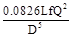(20)

Eqn. (20) may be simplified as eqn. (21)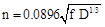(21)

4.2.2. Development of Relation between Scobey and Darcy Weisbach Coefficients

To develop relation between Scobey and Darcy Weisbach friction coefficients, friction losses as per Scobey relation (eqn. 19) and Darcy Weisbach relation (eqn. 16) have been compared as shown in eqn. 22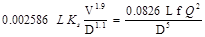(22)

Eqn. (22) may be simplified as eqn. (23)

ks = 20.165 f (Q/D)0.1                         (23)

4.2.3. Comparison of Friction Coefficients Obtained from New Developed Relations

To compare the values of friction coefficients obtained from relations developed in eqn. (21) and eqn. (23), various options of penstock diameter have been considered. Minimum diameter of penstock has been taken as 0.1 m with increment in diameter as 0.1 m upto penstock diameter of 0.5 m then increment of 0.5 m upto penstock diameter of 8.0 m. The penstock discharges have been computed corresponding to all these diameters by taking the economic penstock flow velocity as 3.5 m/s.

Darcy Weisbach friction coefficient has been calculated as per Swami and Jain relation (eqn. 17). The average height of roughness in steel penstock has been taken for as 0.045 mm. Manning and Scobey friction coefficients have been computed as per eqn. (21) and eqn. (23) respectively. All these friction coefficients have been compared in Table 3 and it may be seen that as penstock diameter increases from 0.1 m to 8.0 m, Darcy Weisbach coefficient decreases from 0.0175 to 0.008 while Manning friction coefficient increases from 0.008075 to 0.011334. The values obtained for Manning coefficient are in conformity with the values given for this coefficient in para 4.4.1.2 of Indian Standard (IS) 11625 as 0.008 to 0.012. While carrying out various trials to compute the optimum diameter of penstock, average value of Manning friction coefficient is generally taken in these trials based on different penstock diameters. This average value of Manning coefficient may over estimate friction loss for smaller diameter pipes and underestimate for larger diameter pipes. However as per Gordon , Manning equation may under estimate the friction loss for smaller diameter pipes. With regard to Scobey friction coefficient, it may be further seen from Table 3 that as penstock diameter increases, this coefficient varies (decreases or increases) at randomly. Further, the average value for this coefficient is generally taken as 0.32 which underestimates the friction loss in penstock as minimum value of Scobey coefficient is taken as 0.551261.

Table 3. Comparison of Friction Coefficients used in various formulae.

 Sl. No Penstock Diameter D (m) Penstock Discharge Q (m3/s) Relative Roughness (k/D) Reynolds number (106) Darcy Weisbach coefficient (f) Manning coefficient (n) Scobey coefficient (ks) 1 0.1 0.03 0.0004500 0.35 0.01750 0.008075 0.778030 2 0.2 0.11 0.0002250 0.70 0.01500 0.008392 0.714748 3 0.3 0.25 0.0001500 1.05 0.01450 0.008828 0.719513 4 0.4 0.44 0.0001125 1.40 0.01350 0.008936 0.689443 5 0.5 0.69 0.0000900 1.75 0.01150 0.008560 0.600556 6 1.0 2.75 0.0000450 3.50 0.01000 0.008960 0.559704 7 1.5 6.18 0.0000300 5.25 0.00980 0.009490 0.571207 8 2.0 10.99 0.0000225 7.00 0.00950 0.009803 0.569882 9 2.5 17.17 0.0000180 8.75 0.00920 0.010012 0.564339 10 3.0 24.73 0.0000150 10.50 0.00900 0.010208 0.562229 11 3.5 33.66 0.0000129 12.25 0.00890 0.010416 0.564619 12 4.0 43.96 0.0000113 14.00 0.00880 0.010590 0.565779 13 4.5 55.64 0.0000100 15.75 0.00850 0.010614 0.552966 14 5.0 68.69 0.0000090 17.50 0.00845 0.010770 0.555536 15 5.5 83.11 0.0000082 19.25 0.00840 0.010910 0.557537 16 6.0 98.91 0.0000075 21.00 0.00835 0.011037 0.559062 17 6.5 116.08 0.0000069 22.75 0.00830 0.011151 0.560180 18 7.0 134.63 0.0000064 24.50 0.00820 0.011222 0.557548 19 7.5 154.55 0.0000060 26.25 0.00810 0.011282 0.554561 20 8.0 175.84 0.0000056 28.00 0.00800 0.011334 0.551261

4.3. Determination of Other Head Losses in Penstock (h0)

The other head losses at specials comprises of intake loss (hi), gate/valve loss (hg), bend loss (hb), bi/trifurcation loss (hy), inlet valve loss (hg) and transition piece loss (htr). All these losses can be represented as kav2/2g where v is the penstock flow velocity and ka is the loss coefficient corresponding to that component.

Loss coefficient for penstock intake (ki) depends upon shape of intake mouth. Loss coefficient for gate/valve (kg) and control valve (kv) depend upon the percentage reduction in flow area due to flap of closing device. Loss coefficient for bend (kb) depends upon no. of bends, shape of bends, deflection angle and ratio of radius of bend with diameter of pipe. Loss coefficient at wye (ky) is governed by angle of bi/trifurcation, ratio of cross-sectional area, type and shape of bi/trifurcation. The head loss in transition piece (htr) is represented as ktr(v12-v22)/2g where v1 is penstock velocity or velocity in transition piece (whichever is higher) and v2 is remaining velocity. By substituting v1 and v2 in terms of penstock velocity as k1v2/2g and k2v2/2g respectively, head loss due to transition piece may be taken as ktrev2/2g where ktre is effective head loss coefficient due to transition piece. The other head losses (ho) at specials may be computed from eqn. 24.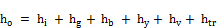(24)

Putting the values of head losses at specials in terms of kav2/2g in eqn. (24), ho can be obtained from eqn. (25)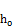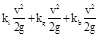(25)

By taking v2/2g as common and writing sum of remaining terms as ko, eqn. (25) may be written as shown in eqn. (26)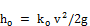(26)

4.4. Determination of Total Head Loss in Penstock (Thl)

The total head loss (Thl) can be obtained as per eqn. 27.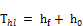(27)

By putting value of hf from Darcy Weisbach relation (eqn. 15) and ho from eqn. (26) in eqn.(27), Thl can be obtained from eqn. (28)

Thl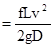+ ko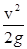(28)

By taking v2/2g common in eqn. (28), remaining terms shall be constant. Substituting sum of remaining terms as ktl, Thl can be obtained from eqn. (29)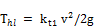(29)

4.5. Development of Relation between Total Head Loss and Friction Loss

The total head loss comprises of friction loss and losses at specials and depends on diameter and length of penstock. For projects with high gradient, length of penstock required will be small. For projects having flatter gradient, penstock length is longer. As the length of penstock increases, total head loss in penstock also increases. The penstock can be categorized as longer and shorter penstock on the basis of the ratio (Rplh) of penstock length and gross head. For longer penstock, the main head loss is due to friction. For smaller penstock, the contribution of friction loss in total head loss is less. Therefore, ratio of total head loss and friction loss (Rthf ) for longer penstock will be less and for shorter penstock, will be more. To study the variation in contribution of friction loss in total head loss corresponding to variation in length of penstock, the values of Rplh and Rthf for various hydro electric projects have been computed and shown in Table 4

Table 4. Friction and Total Head Losses in Penstock of Hydroelectric Projects.

 Sl. No. Project Name Head Loss (m) Ratio of penstock length/Gross head (Rplh) Ratio of Total head loss and Friction loss (Rthf) Friction Others (specials) Total 1 Pemashelpu 0.54 0.84 1.38 1.21 2.55 2 Debra 0.24 0.35 0.59 1.45 2.46 3 Kotijhala 0.25 0.37 0.62 1.49 2.45 4 Divri 0.33 0.47 0.79 1.62 2.41 5 Sarbari-ii 0.73 0.98 1.72 1.91 2.34 6 Dhera 0.29 0.39 0.68 1.99 2.32 7 Nyikgong 0.18 0.24 0.41 2.00 2.32 8 Gaundar 0.22 0.28 0.50 2.14 2.29 9 Ditchi 1.12 1.42 2.53 2.22 2.27 10 Jirah 2.88 3.62 6.50 2.29 2.26 11 Wachham 0.20 0.25 0.46 2.45 2.23 12 Luni-II 3.04 3.63 6.67 2.68 2.19 13 Gaj 0.17 0.19 0.35 3.15 2.13 14 Luni-III 4.11 4.48 8.58 3.44 2.09 15 Kuti 0.46 0.48 0.94 3.74 2.06 16 Baram 1.58 1.44 3.01 5.49 1.91 17 Dugtu 1.02 0.68 1.70 11.52 1.66 18 Keyi 2.81 1.76 4.56 12.96 1.63 19 Phunchung 7.52 3.03 10.54 28.08 1.40 20 Thru 17.08 5.04 22.12 42.75 1.30 21 Kamlang 2.98 0.76 3.75 50.30 1.26

From the analysis of results shown in Table 4, it may be seen that for shorter penstock (Rplh as 1.21) and longer penstock (Rplh as 50.30), ratio of total head loss with friction loss (Rthf) is 2.55 and 1.26 respectively. It means that contribution of other losses in total head loss for longer penstock may be as less as 26% of friction loss while for shorter penstock, this contribution may be as high as 155% of friction loss. The variation of Rthf and Rplh for various hydro electric projects has been shown in fig 5. The best fit curve has been drawn between values of Rthf and Rplh based on regression analysis. The equation of best fit curve is shown in eqn. (30).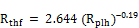(30)

The correlation coefficient (R2) for the above relation has been observed as 0.837 which is within permissible limit.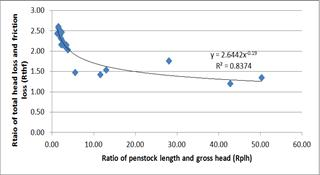Fig. 5. Relation of Rthf   and Rplh.

Therefore, relation shown in eqn. (30) may be used for hydro electric projects. By placing Rthf as Thl/hf and Rplh as L/H in eqn. (30), the resulting eqn. is shown as eqn. (31)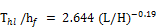(31)

For any project, the length of penstock and gross head are site specific parameters. These will remain same while carrying out various trials in order to determine optimum diameter of penstock. Therefore, term on right hand side of eqn. (31) shall be constant. By replacing right hand side in eqn. (31) as constant (kt ), the resulting eqn. is shown as eqn. (32).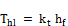(32)

5. Development of New Relation for Optimum Design of Penstock Based on Total Head Loss

The available analytical relation for optimum design of penstock is based on minimizing the annualized cost of penstock system considering only friction loss. In this study, a new relation has been developed for optimum design of penstock considering losses at specials in addition to friction loss. The steps followed are as follows

Computation of annualized cost of penstock

Computation of annual loss of revenue due to energy loss caused by total head loss

Development of relation for optimum diameter of penstock based on total head loss

5.1. Determination of Annualized Cost of Penstock (Ep)

Penstocks are laid on surface or buried/embedded in ground. The surface penstocks are laid above ground and supported on concrete blocks at regular interval. Buried penstocks are laid in tunnel or cut and fill section which requires excavation of trench/tunnel of slightly higher size than that of penstock so that penstock may be laid inside. After laying penstock in tunnel, concreting is done in extra portion. For surface penstock, the cost of excavation as well as concrete lining will be less. Total expenditure on penstock will comprise of cost of excavation for laying penstock, cost of concrete lining and cost of penstock.

5.1.1. Cost of Excavation for Laying Penstock (Cex )

For laying the penstock, trench/tunnel of higher diameter (De + 0.33De) is excavated. Cost of excavation for laying penstock is computed by multiplying volume of material excavated with unit rate of excavation as per eqn. (33).

Cex =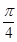(De + 0.33 De)2 Ce                      (33)

After simplifying, eqn. (33) may be written as per eqn. (34)(34)

5.1.2. Cost of Concrete Lining (Ccl )

The cost of concrete lining for penstock may be computed by multiplying the quantity and unit rate of concrete as per eqn. (35). For computation of quantity, thickness of concrete lining may be taken as 0.165 De.(35)

After simplifying, eqn. (35) may be written as per eqn. (36)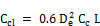(36)

5.1.3. Cost of Penstock (Csp)

To compute cost of the penstock, the weight of penstock is required which can be computed in terms of penstock thickness, weight of penstock stiffeners as ratio (i) of penstock weight and density of penstock material (r) as per eqn. (37)(37)

The penstock thickness can be computed in terms of water pressure (pr) developed in pipe due to gross head (Hgross), permissible stresses in pipe material, joint efficiency and other parameters as shown in eqn. (38)

t =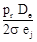(38)

In case of sudden closure of the turbine, water pressure/head inside the penstock increases due to water hammer. Taking increase in head as ΔH, total design head inside the pipe (H) becomes (Hgross + ΔH). By replacing pr in eqn. (38) as 0.1H, t can be computed from eqn. (39)

t =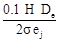(39)

Thickness computed from eqn. (39) should be more than the minimum handling thickness (tmin) of the penstock which is equal to (De + 500)/400 where De and tmin are in mm. ΔH can be computed in terms of velocity of pressure wave and other parameters as shown in eqn. (40)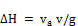(40)

velocity of pressure wave can be computed in terms of Bulk modulus of fluid and Young modulus of pipe material as per eqn. (41)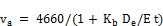(41)

By placing the value of t from eqn. (39) in eqn. (37), Wg may be computed from the eqn. (42)

Wg =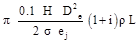(42)

cost of penstock (Csp) may be computed by multiplying penstock weight as per eqn. (42) with unit cost of pipe material and dividing by 9.81 to put the value ofin MPa as shown in eqn. (43). The value of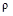has been taken as 7850 kg/m3,

Csp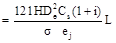(43)

5.1.4. Determination of Total Expenditure on Penstock (Texp)

Total expenditure on penstock is computed by adding cost of excavation, cost of concreting and cost of penstock as per eqn. (44).(44)

Putting the values of Cex, Ccl and Csp from eqn. (34), eqn. (36) and eqn. (43) respectively in eqn. (44), Texp may be computed as per eqn. (45).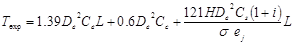(45)

5.1.5. Annualized Cost of Penstock (Ep)

Annual expenditure on penstock may be taken as some percentage (p) of Texp. Therefore, p is taken as the ratio of annual expenditure and total expenditure on penstock. Ep is computed by multiplying Texp as per eqn. (45) and p as shown in eqn. (46)

Ep= De 2 [1.39 Ce + 0.6Cc] p L     (46)

5.2. Computation of Annual Loss of Revenue due to Energy loss caused by total Head Loss

To compute annual loss of revenue due to total head loss, the power loss (Pl) due to this head loss is required which is computed in terms of discharge, total head loss, and plant efficiency as shown in eqn. (47)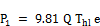(47)

The annual energy loss (El) can be computed by multiplying Pl as per eqn. (47) with total hours of operation in the year (hr). hr is computed by multiplying plant power factor (Pf) and total hours (8760) in a year. El is computed as per eqn. (48)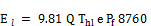(48)

The annual loss of revenue (Et) due to energy loss can be computed by multiplying El as per eqn. (48) and cost of power (Cp) as shown in eqn. (49)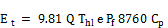(49)

5.3. Development of Relation for Optimum Diameter of Penstock Based on Total Head Loss

In order to get the optimized penstock diameter (De) of penstock, the annualized penstock cost (Ep + Et) is to be differentiated with respect to penstock diameter and equated to zero as shown in eqn. (50)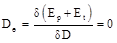(50)

Et can be obtained by putting the value of Thl from eqn. (32) in eqn. (49) as shown in eqn. (51)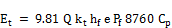(51)

value of hf in eqn. (51) may be put up from eqn. (16) as shown in eqn. (52)

Et = 9.81Q kt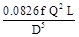* e  Pf 8760 Cp(52)

Placing the value of Ep and Et in eqn. (50), from eqn. (46) and eqn. (52) respectively, the resulting eqn. is shown in eqn. (53)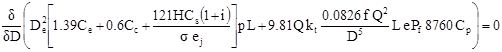(53)

By differentiating eqn. (53), the relation obtained is shown in eqn. (54).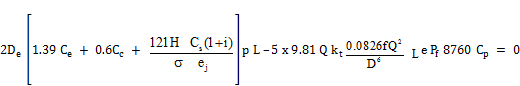(54)

After simplifying, eqn. (54) may be written as eqn. (55)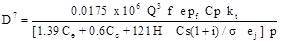(55)

Putting the value of kt in eqn. (55) from eqn. (31), the relation obtained is shown in eqn. (56).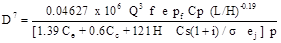(56)

The relation shown in eqn. (56) is the new relation for optimum diameter of penstock based on total head loss

6. Applicability of Developed Relation for Hydro Electric Project

The relation developed in eqn. (56) has been used to determine the optimum penstock diameter of various HE Projects.

To compute the optimum penstock diameter, the values of parameters Cp, Ce, Cc and Cs have been taken as INR 5.5 per unit rate of electricity generation, INR 5150 per m3, INR 8000 per m3 and INR 100 per kg respectively. The values of parameters e, Pf σ, ej and p have been taken as 0.85, 0.5 183.33 MPa, 1.0 and 0.16 respectively. The optimum diameter of penstock calculated for each project has been shown in Table 5. The saving in annual cost of each penstock may be seen in this table. The saving in cost is higher for penstock having lower ratio of penstock length with gross head. This saving decreases as the ratio of penstock length and gross head increases.

Table 5. Penstock Diameter and Annual Cost Saving As Per Developed Method for various HE projects.

 Sl. No. Project Diameter as provided at site/DPR (m) Diameter as per new developed relation (m) % Increase in Diameter Annual Cost of penstock ( INR million ) as per site / DPR as per new developed method Net Saving In % of cost of penstock 1 Pemashelpu 3.11 3.56 14.31 36.33 32.80 3.529 9.714 2 Debra 1.01 1.14 13.75 0.84 0.77 0.076 9.044 3 Kotijhala 0.58 0.66 13.67 0.23 0.21 0.020 8.939 4 Divri 1.22 1.38 13.41 1.85 1.69 0.160 8.639 5 Sarbari-ii 1.27 1.43 12.90 4.75 4.36 0.382 8.048 6 Dhera 1.07 1.20 12.77 1.09 1.00 0.086 7.892 7 Nyikgong 2.82 3.18 12.76 6.77 6.24 0.534 7.882 8 Gaundar 0.53 0.60 12.56 0.14 0.13 0.011 7.655 9 Ditchi 0.86 0.97 12.44 2.77 2.56 0.208 7.528 10 Jirah 0.79 0.89 12.35 6.55 6.07 0.486 7.418 11 Wachham 0.91 1.02 12.14 0.47 0.44 0.034 7.188 12 Luni-II 0.87 0.97 11.87 8.27 7.70 0.570 6.896 13 Gaj 1.55 1.73 11.38 1.28 1.20 0.082 6.377 14 Luni-III 0.87 0.97 11.11 10.82 10.16 0.660 6.098 15 Kuti 0.53 0.59 10.86 0.26 0.25 0.015 5.842 16 Baram 1.00 1.09 9.71 4.19 3.99 0.198 4.737 17 Dugtu 0.38 0.41 7.53 0.202 0.196 0.006 2.922 18 Keyi 2.68 2.87 7.18 66.92 65.13 1.789 2.674 19 Phunchung 3.41 3.58 4.96 278.61 274.94 3.672 1.318 20 Thru 3.34 3.47 3.77 577.00 572.48 4.513 0.782 21 Kamlang 4.64 4.79 3.31 178.57 177.47 1.094 0.613

7. Conclusion

The various relations available for optimum design of penstock have been compared and it was observed that these relations provide different values of optimum penstock diameter resulting in different cost. Some of these relations are based on minimizing annual cost of penstock considering friction loss only whereas in practice other losses in penstock also occurs and needed to be considered. In addition, the different relations available for friction loss also provides different values of losses in penstock. A new method has been developed to optimize the design of penstock for hydro power projects on the basis of minimizing annual project cost considering total head loss (friction and other losses). All these losses have been formulated using Darcy Weisbach formula. The newly developed relation has been used for 21 hydro power projects with capacity ranging from 25 kW to 60 MW to find out the optimum diameter and compared with results obtained as provided at site/DPR. By providing penstock diameter as per new method, though the penstock diameter increased in the range of 3.31 to 14.31%, it resulted in the net saving in annual cost of penstock. The saving has been obtained from 0.613 % to 9.714% of earlier penstock cost which justify the applicability of the new method for optimum design of penstock for hydro power projects.

Symbols Used

r = density of penstock material

n = Manning’s roughness coefficient

σ = permissible stress in penstock

a = cost of pipe in \$ per lb

b = cost of 1 kWh of energy in \$

C = capital cost of penstock installed per unit weight

Cp = cost of 1 kWh of energy

Ccl = cost of concrete lining / cum

Cc = unit rate of concrete lining

Ce = cost of excavation / cum for laying penstock

Cex = cost of excavation per unit length of penstock

Cs = cost of steel / kg

Csp = cost of penstock

D = Diameter of penstock

De = economic diameter of penstock

e = turbine/generator efficiency

ej = joint efficiency of penstock

E = Young modulus of elasticity of steel

El = annual loss of energy due to total head loss

Ep = annualised cost of penstock

Et = annual loss of revenue

f = friction factor in Darcy Weisbach and ASCE relation

l = loss factor in Voetsch and Fresen relation

h = annual hours of operation

hi..tr= head loss at penstock components. i..tr denotes the respective component

ho = other head losses in penstock

hf = friction loss in penstock

HE = hydro electric

i = ratio of weight of stiffeners and weight of penstock

int = interest rate in percentage

k = average roughness in penstock

khl = coefficient of total head loss in penstock

kitr= coefficient of head loss at penstock component. itr denotes the respective component

ko = coefficient of other head losses in penstock

ks = Scobey friction factor

K = coefficient in Voetsch and Fresen

Kb = Bulk modulus of elasticity of water

L = length of penstock

M = composite value of power

ns = ratio of weight of stiffeners and weight of penstock

nr. = repayment period

pr = water pressure inside the penstock

p = ratio of annual charges to installation cost of penstock

P = Installed capacity of project

Pl = power loss due to total head loss

Pwf = present worth factor

Q = Penstock discharge

r = ratio of annual charges to installation cost of penstock

Rplh = ratio of penstock length and gross head

Rthf = ratio of total loss and friction loss

S = allowable stress in psi

t = thickness of penstock

Texp= total expenditure on penstock per unit length(INR)

Thl = total head loss in penstock

v = flow velocity in penstock

va = velocity of pressure wave

W = specific weight of steel

Wg = weight of penstock per unit length

INR= Indian Rupees

References

1. Colebrook, C.F. and White, C.M. (1937) "Experiments with Fluid Friction in Roughened Pipes", Proc. Roy. Soc. Series A, 161, 367.
2. Colebrook, C.F. and White, C.M. (1937-38) "The Reduction of Carrying Capacity of Pipes with Age", J. Inst. C.E., 7, 99.
3. Colebrook C.F. (1939) "Turbulent flow in pipes with particular reference to the transition region between the smooth and rough pipe laws", J Inst Civil Engineers, London, Vol. 11, pp. 133-156.
4. Fahlbusch, F.(1982) "power tunnels and penstock the economics re-examined" International Water power and dam construction, Vol. 34 no 6, June 1982, pp 13-15.
5. Fablbusch F (1987), "Determining Diameters for Power Tunnels ad Pressure Shafts" Water Power and Dam Construction February 1987.
6. Indian Standard:11625-1986(2001); "Criteria for Hydraulic Design of Penstock". Bureau of Indian Standards, Delhi PP-16
7. Manning, R. (1891) "On the Flow of Water in Open Channels and Pipes" Trans. Inst. C.E. of Ireland, 20, 161.
8. Moody L.F. (1944) "Friction Factors for Pipe Flow" Transactions of the ASME, Vol. 66(8), pp. 671–684.
9. Nikuradse, J. (1933) "Stroemungsgesetze in rauhen Rohren." Ver. Dtsch. Ing. Forsch., 361, 1933
10. Gordon, J.L., (1978), "Design criteria for exposed hydro penstock" Canadian Journal of civil Engineering, 5, pp, 340 – 351.
11. Sarkaria, G.S. (1957), "Penstocks sized Quickly" Engineering News Record, Aug.15, 1957, pp 78-79.
12. Sarkaria G.S (1979), "Economic Penstock Diameter: A 20-year Review" Water Power and Dam Construction November 1979.
13. ASCE Manuals and Reports on Engineering Practice No. 79, (1993), Steel Penstocks, ASCE, American Society of civil Engineers, New York, 1993
14. Scobey F.C. (1930), " The flow of water in Riveted and analogous pipes", Vol 150, USDA, Washington, DC, 45
15. Swamee P.K. and Jain A.K. (1976) "Explicit equations for pipe-flow problems" Journal of the Hydraulics Division (ASCE), Vol 102 (5), pp. 657–664.
16. Voetsch Charles and Fresen M.H (1938),"Economic Diameter of Steel Penstock" ASCE Transaction Vol 103, paper no 1982.
17. USBR, Engineering Monogram No. 3 (1986), Welded Steel Penstocks, United States Department of The interior Bureau of Reclamation, Washington, 1986.

 Contents 1. 2. 2.1. 2.2. 2.3. 2.4. 3. 4. 4.1. 4.2. 4.3. 4.4. 4.5. 5. 5.1. 5.2. 5.3. 6. 7.
Article ToolsAbstractPDF(397K)